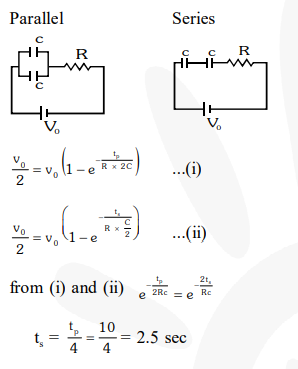# Combination of two identical capacitors, a resistor R and a dc voltage source of voltage 6V is used in an experiment on (C–R) circuit.

Question:

Combination of two identical capacitors, a resistor $\mathrm{R}$ and a dc voltage source of voltage 6V is used in an experiment on $(\mathrm{C}-\mathrm{R})$ circuit. It is found that for a parallel combination of the capacitor the time in which the voltage of the fully charged combination reduces to half its original voltage is 10 second. For series combination the time needed for reducing the voltage of the fully charged series combination by half is :-

1. 20 second

2. 10 second

3. 5 second

4. 2.5 second

Correct Option: , 4

Solution: